### Home > PCT > Chapter Ch4 > Lesson 4.1.3 > Problem4-45

4-45.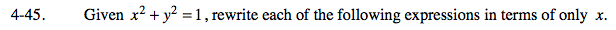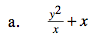$\frac{y^2}{x}+\frac{x^2}{x}$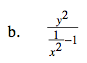$\frac{\frac{y^2}{1}}{\frac{1}{x^2}-\frac{1}{1}}\cdot\frac{\frac{x^2}{1}}{\frac{x^2}{1}}$

$1-x^2=y^2$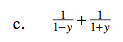$\frac{1+y}{(1-y)(1+y)}+\frac{1-y}{(1-y)(1+y)}$

$1-y^2=x^2$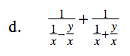$\small \frac{1}{\frac{1-y}{x}}+\frac{1}{\frac{1+y}{x}}$

$\frac{x}{1-y}+\frac{x}{1+y}$